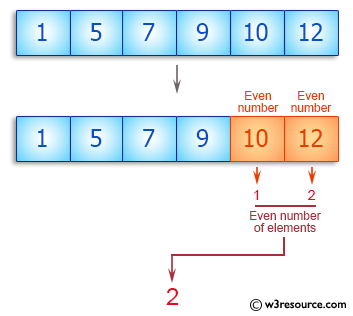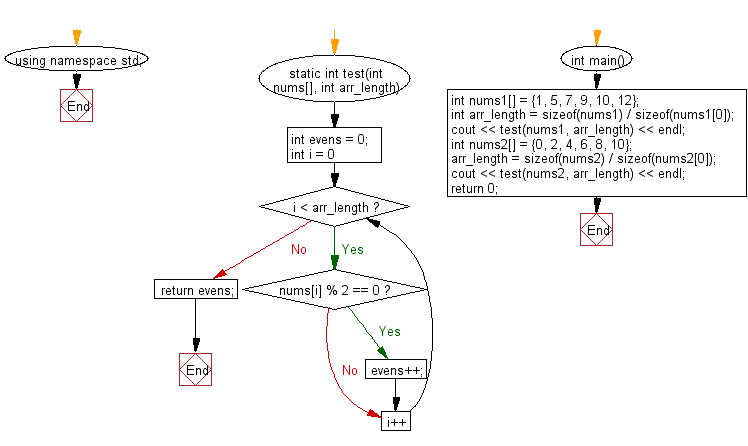﻿ C++ : Count even number of elements in an array# C++ Exercises: Count even number of elements in a given array of integers

## C++ Basic Algorithm: Exercise-98 with Solution

Write a C++ program to count even number of elements in a given array of integers.

Sample Solution:

C++ Code :

``````#include <iostream>
using namespace std;

static int test(int nums[], int arr_length)
{
int evens = 0;

for (int i = 0; i < arr_length; i++)
{
if (nums[i] % 2 == 0) evens++;
}
return evens;
}

int main()
{
int nums1[] = {1, 5, 7, 9, 10, 12};
int arr_length = sizeof(nums1) / sizeof(nums1);
cout << test(nums1, arr_length) << endl;
int nums2[] = {0, 2, 4, 6, 8, 10};
arr_length = sizeof(nums2) / sizeof(nums2);
cout << test(nums2, arr_length) << endl;
return 0;
}
``````

Sample Output:

```2
6
```

Pictorial Presentation:Flowchart:C++ Code Editor: